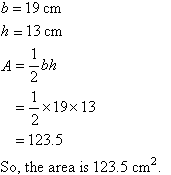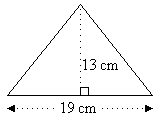Year 10 Interactive Maths - Second Edition

## Area

Area is the size of the region enclosed within a plane figure. To the carpenter or carpet layer, who is concerned with the amount of material to be used, measurement of area is very important.

Area is measured in square units.  The most frequently used units of area are mm2, cm2 and m2.  Land areas are often given in hectares (ha).

1 ha = 10 000 m2

Areas of the following figures are often required to solve real world problems.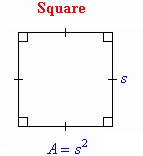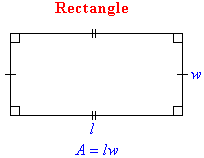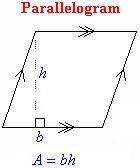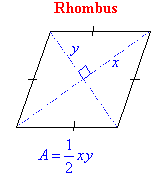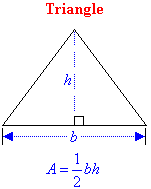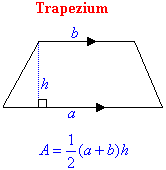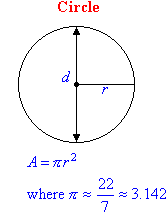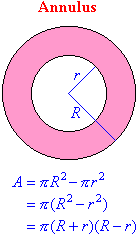## Area of a Square

###### Recall that:
A square with sides of length s units has an area of A square units given by the formula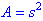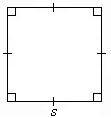#### Example 13

Find the area of a square flower bed of side 7.2 m.

##### Solution: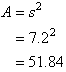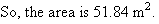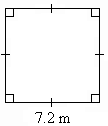###### Note:

To find the area of a region enclosed within a plane figure, draw a diagram and write an appropriate formula. Then substitute the given values and use a calculator, if necessary, to obtain the required area.

## Area of a Rectangle

###### Recall that:
A rectangle with length l units and width w units has an area of A square units given by the formula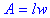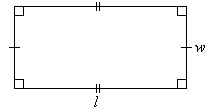#### Example 14

Find the area of a rectangular field 45 m long and 38 m wide.

##### Solution: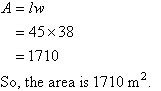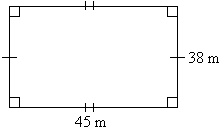## Area of a Triangle

###### Recall that:
A triangle with base b units and height h units has an area of A square units given by the formula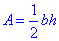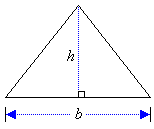#### Example 15

Find the area of a triangle with base 19 cm and height 13 cm.

##### Solution: